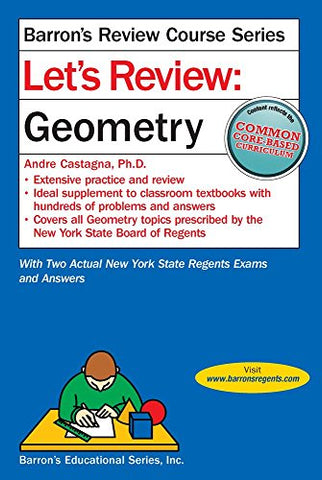# Let's Review Geometry (Let's Review Series)

• ISBN-13: 9781438007021
• \$11.30

Only 5 left!

This brand new book includes the most up-to-date information on the Geometry Common Core Regents Exam. Students can use this review guide to prepare for their Geometry Regents Exams. Inside, separate chapters explain and provide practice problems on: the language of geometry, basic geometric relationships (parallel lines, polygons, and triangle relationships), constructions, an introduction to geometric proof transformations, triangle congruence, similarity and right triangle trigonometry, parallelograms, circles and arcs, coordinate geometry and proofs on the coordinate plane, and volume (modeling 3-D shapes in practice applications). Includes the first two actual Regents exams in Geometry, plus answer keys for each test.